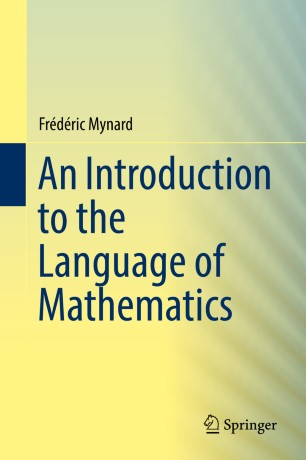# An Introduction to the Language of Mathematics

• Frédéric MynardTextbook

1. Front Matter
Pages i-xii
2. Frédéric Mynard
Pages 1-49
3. Frédéric Mynard
Pages 51-94
4. Frédéric Mynard
Pages 95-129
5. Frédéric Mynard
Pages 131-143
6. Back Matter
Pages 145-185

### Introduction

This is a textbook for an undergraduate mathematics major transition course from technique-based mathematics (such as Algebra and Calculus) to proof-based mathematics. It motivates the introduction of the formal language of logic and set theory and develops the basics with examples, exercises with solutions and exercises without. It then moves to a discussion of proof structure and basic proof techniques, including proofs by induction with extensive examples. An in-depth treatment of relations, particularly equivalence and order relations completes the exposition of the basic language of mathematics. The last chapter treats infinite cardinalities. An appendix gives some complement on induction and order, and another provides full solutions of the in-text exercises.

The primary audience is undergraduate mathematics major, but independent readers interested in mathematics can also use the book for self-study.

### Keywords

propositional logic set theory proofs mathematical induction relations equivalence relation order relation infinite cardinality

#### Authors and affiliations

• Frédéric Mynard
• 1
1. 1.MathematicsNew Jersey City UniversityJersey CityUSA